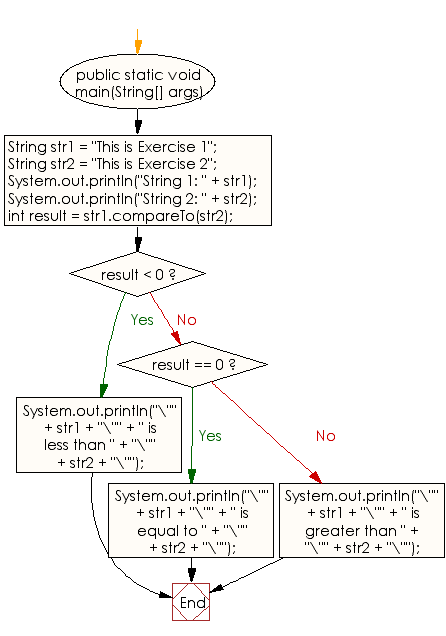﻿ Java exercises: Compare two strings lexicographically - w3resource# Java String Exercises: Compare two strings lexicographically

## Java String: Exercise-5 with Solution

Write a Java program to compare two strings lexicographically. Two strings are lexicographically equal if they are the same length and contain the same characters in the same positions.

Sample Solution:

Java Code:

``````public class Exercise5 {
public static void main(String[] args)
{
String str1 = "This is Exercise 1";
String str2 = "This is Exercise 2";

System.out.println("String 1: " + str1);
System.out.println("String 2: " + str2);

// Compare the two strings.
int result = str1.compareTo(str2);

// Display the results of the comparison.
if (result < 0)
{
System.out.println("\"" + str1 + "\"" +
" is less than " +
"\"" + str2 + "\"");
}
else if (result == 0)
{
System.out.println("\"" + str1 + "\"" +
" is equal to " +
"\"" + str2 + "\"");
}
else // if (result > 0)
{
System.out.println("\"" + str1 + "\"" +
" is greater than " +
"\"" + str2 + "\"");
}
}
}
```
```

Sample Output:

```String 1: This is Exercise 1
String 2: This is Exercise 2
"This is Exercise 1" is less than "This is Exercise 2"
```

Flowchart:Visualize Java code execution (Python Tutor):

Java Code Editor:

Improve this sample solution and post your code through Disqus

What is the difficulty level of this exercise?

﻿

## Java: Tips of the Day

Array vs ArrayLists:

The main difference between these two is that an Array is of fixed size so once you have created an Array you cannot change it but the ArrayList is not of fixed size. You can create instances of ArrayLists without specifying its size. So if you create such instances of an ArrayList without specifying its size Java will create an instance of an ArrayList of default size.

Once an ArrayList is full it re-sizes itself. In fact, an ArrayList is internally supported by an array. So when an ArrayList is resized it will slow down its performance a bit as the contents of the old Array must be copied to a new Array.

At the same time, it's compulsory to specify the size of an Array directly or indirectly while creating it. And also Arrays can store both primitives and objects while ArrayLists only can store objects.

Ref: https://bit.ly/3o8L2KH